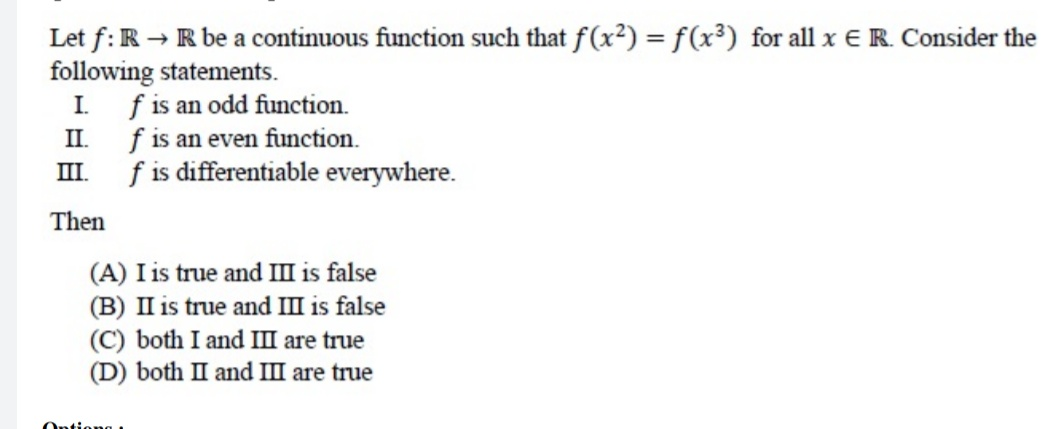# Doubt from FunctionsWe have f(-x^3)=f(x^2) = f(x^3) and since y=x^3 is onto we can see that in general f(-z)=f(z), \ \forall \ z \in \mathbb{R}. Hence f(x) is even.

Now we have f(a) = f\left(a^\dfrac{2}{3}\right)=\cdots= f\left(a^\dfrac{2^n}{3^n}\right)=f(1) since \left(\dfrac{2}{3} \right)^n \rightarrow 0 and using continuity.

We also have for 0<b<1, f(b) = f\left(b^\dfrac{3}{2}\right) = \cdots = f\left(b^\dfrac{3^n}{2^n}\right) = f(0)

So if it has to be a function we need f(0)=f(1) and hence f is a constant function.

1 Like